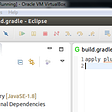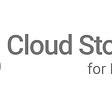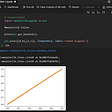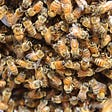# Automate ODK survey forms using XLSform, Python or R

Example: Ask these set of questions to

1. Please select a possible spare parts for (__machine_name___) that can be manufactured in Nepal? (Multi select)
2. How many (__machine__name___) do you own? (Integer)
3. Are are the (__machine__name__) in working condition? (select one)

machine_name = combine harvester’,’diesel minitiller’,’diesel pump’, ‘disc harrow’, ‘paddy thresher’, ‘petrol minitiller’,’power tiller’,’pump set’,’reaper’,’rice mill’,’rotavator’, ‘thresher rice’, ‘thresher wheat’,’tractor’, ‘water_pump’, ‘thresher’

Copy-pasting these questions for each of the machines in xlsform is extremely time-consuming. And therefore, this solution somewhat automates the process.

USING XLSFORM:

The first and the go-to method would be using the begin-repeat function within xlsform. This would be the goto method as most of the question is being built using xlsform already.

Survey Sheet:

Choices sheet

USING Python:

1. Import pandas library. If not installed, quickly install the Pandas library using
2. Define the column names required for the excel sheet. The mandatory columns type, name, label must be explicitly defined. The other column headings such as relevant, constraint, appearance and calculation can be added as required. For this example, appearance column has been added.
3. Create an empty dataframe, defining the columns as the earlier set col_names. Also define all the machine names that the questions need to be asked for.
`#1pip install pandas#2col_names =  ['type', 'name', 'label','appearance']#3df_1 =   pd.DataFrame(columns = col_names)`

4. Loop through each of the machines and use the .loc function to create the survey questions for each machine.

`#add new row to end of DataFramefor machine in machines:## type columndf_1.loc[len(df_1.index)] = ['begin group',f'Group_start_{machine}', f'{machine } group','field_list']df_1.loc[len(df_1.index)] = [f'select_multiple spare_parts_{machine}',f'spare_parts {machine}' , f'Please select a possible spare parts for {machine }that can be manufactured in Nepal','compact']df_1.loc[len(df_1.index)] = ['decimal', f'total_machines {machine}' , f'How many {machine } do you own?','']df_1.loc[len(df_1.index)] = [f'select_one {machine}_working', f'{machine}_working' , f'Are are the {machine } in working condition?','']df_1.loc[len(df_1.index)] = [f'end group',f'Group_End_{machine}' , f'{machine } end group','']## empty row for cleaner excel filedf_1.loc[len(df_1.index)] = ['','' , '','']`
• Apologies for no indentations in python code

5. Finally, export the dataframe as Excel file. you might have to install openpyxl

`pip install openpyxldf_1.to_excel('Automate xlsform questions/quick_file_2.xlsx', index = False, sheet_name = 'survey')`

Using R

1. Install the package “glue” using install.package(‘glue’) for using a function that is similar to python’s F function.
2. The steps are pretty similar to the Python code
`library(glue)machines =  c('combine_harvester','diesel_minitiller','diesel_pump', 'disc_harrow','paddy_thresher','petrol_minitiller','power_tiller','pump_set','reaper','rice_mill','rotavator', 'thresher_rice','thresher_wheat','tractor', 'wate_pump', 'thresher')#create data frame with 0 rows and 3 columnsdf <- data.frame(matrix(ncol = 4, nrow = 0))#provide column namescolnames(df) <- c('type', 'name', 'label','appereance')#iterate through each of the machines to add questionsfor (machine in machines) {df[nrow(df) + 1,] = c('begin group', glue('{machine}_group'),glue('{machine} Group'),'field_list')df[nrow(df) + 1,] = c(glue('select_multiple spare_parts_{machine}'), glue('spare_parts_{machine}'),glue('Please select a possible spare parts for {machine }that can be manufactured in Nepal'),'compact')df[nrow(df) + 1,] = c('decimal', glue('total_machines {machine}'),glue('total_machines {machine }'),'')df[nrow(df) + 1,] = c(glue('select_one {machine}'), glue('{machine}_working'),glue('Are are the {machine } in working condition?'),'')df[nrow(df) + 1,] = c('end group', glue('{machine}_end_group'),glue('{machine} End Group'),'')}# Export the dataframe to excel file`

Your xlsform is ready. The hassle of having to copy/paste and rename the machine name for each question is now history.

All of the three codes can be found here. https://github.com/Saralkarki/automate_xlsform_questions

please feel free to contribute and suggest feedback where the code and the thought process can be optimized.

--

--

--

## More from weirdbutwired

Time to write…

Love podcasts or audiobooks? Learn on the go with our new app.

## Weekly coding & goals progress [Week 34] 🚀## Getting to know Gradle — Using## AWS Cloud Practitioner completed — what’s next?## Random Paths in Gamedev Beatdown## Writing Scalable API is like making Khichdi## Emotions management when pair programmingTime to write…

## Visualization Package/Library for Missing Data in Python & R## Data Visualization Techniques for Data Science You must know!!## %matplotlib inline## Why you should use Swarmplots for Data Visualization